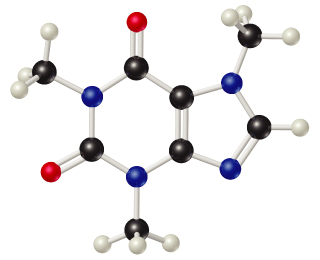# Problem: Caffeine (C8H10N4O2) is a stimulant found in coffee and tea. A solution of caffeine in the solvent chloroform (CHCl3) has a concentration of 0.0510 m.Calculate the mole fraction of caffeine in the solution.

###### FREE Expert Solution
95% (272 ratings)
###### FREE Expert Solution

We’re being asked to calculate for the mole fraction of caffeine (C8H10N4O2in the solution.

The solution is made up of caffeine (C8H10N4O2) and chloroform (CHCl3) with a concentration of 0.0510 m. Since chloroform is the solvent, we can say that caffeine is the solute.

Mole Fraction (X) relates the moles of solute and solvent within a solution:

We’ll calculate the mole fraction of caffeine using the following steps:

95% (272 ratings)###### Problem Details

Caffeine (C8H10N4O2) is a stimulant found in coffee and tea. A solution of caffeine in the solvent chloroform (CHCl3) has a concentration of 0.0510 m.Calculate the mole fraction of caffeine in the solution.

What scientific concept do you need to know in order to solve this problem?

Our tutors have indicated that to solve this problem you will need to apply the Mole Fraction concept. You can view video lessons to learn Mole Fraction. Or if you need more Mole Fraction practice, you can also practice Mole Fraction practice problems.

What is the difficulty of this problem?

Our tutors rated the difficulty ofCaffeine (C8H10N4O2) is a stimulant found in coffee and tea....as medium difficulty.

How long does this problem take to solve?

Our expert Chemistry tutor, Sabrina took 6 minutes and 24 seconds to solve this problem. You can follow their steps in the video explanation above.

What professor is this problem relevant for?

Based on our data, we think this problem is relevant for Professor Mohseni's class at East Tennessee State University.